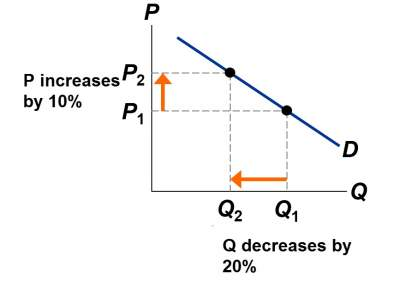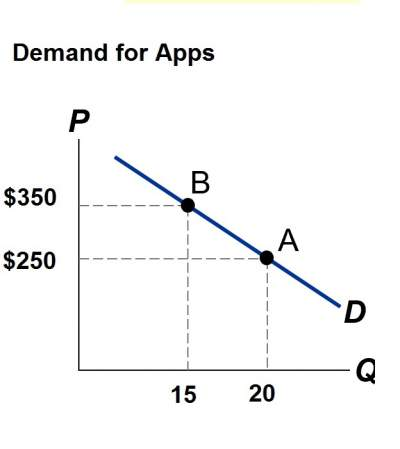# Introduction to Elasticity

## Definition

Elasticity is a numerical measure of the responsiveness of Qd or Qs to one of its determinants.

So basically, elasticity is about measuring the effect of one variable onto another variable.
For example, if a company specializes in developing applications for mobile devices. If this company decides to increase the price for their services then what would be the impact on demands.

## Price Elasticity of Demand

Price Elasticity of Demand = (Percentage change in Qd) / (Percentage change in P)

• Price elasticity of demand measures how much Qd responds to a change in P.
• This measures the price-sensitivity of buyers' demand.

### Example

Assume that price elasticity of demand = 20% / 10% = 2. See the figure below:Note that along a D curve, P and Q are supposed to move in opposite directions, which would make price elasticity negative. However, for the sake of simplicity, the minus sign is ignored and consider that price elasticities as positive numbers.

### Calculating Percentage Changes

Standard method of computing the percentage (%) change is given below:
(end value − start value) / (start value) × 100%

So, for example, assuming the company that develops apps, and wanting to increase the price (depicted in the figure below as moving from A to B).
The % change in P = (\$350 − \$250) /\$250 = 40%The problem with standard method is that it provides different answers depending on the starting point.
From A to B, P increases 40%, Q falls 25%, elasticity = 25/40 = 0.62
From B to A, P decreases 28%, Q increases 25%, elasticity = 25/28 = 0.89

That's the reason why another method is used and it's called midpoint method, which is represented by the following formula:
(end value − start value) / (midpoint) × 100%

• The midpoint represents the average of end value and start value.
• In this case, whichever is used as start or end wouldn't change the result.

#### Example

• Using the midpoint method, the percentage change in P is as follows:
(\$350 − \$250) / (\$300) × 100% = 33.33%
• The percentage in Q would be:
(20 − 15) / (17.5) × 100% = 28.57 %
• The price elasticity of demand is calculated as:
28.57 / 33.33 = 0.86

### Exercise

Exercise on Calculating the Elasticity

Check your answers here: Solution to the Exercise on Calculating the Elasticity

### What determines price elasticity?

We will consider a couple of examples help to understand the determines price elasticity, where each one compares two common goods.
In each example:
• Assume that the prices of both goods increase by 25%.
• The particular good for which Qd falls the most (in percent) has the highest price elasticity of demand. Which good is it? And Why?
• What findings do we draw from each example about the determinants of the price elasticity of demand?

#### Example 1: Aspirin versus Jetski Rides

• The prices of both of these goods rise by 25%.
For which good does Qd drop the most? Why?
• A rise in the price of Aspirin wouldn't cause any effect on its demand. Millions of people use Aspirin because, on the other hand
• If the price of a Jetski ride rises, some people may not be obliged to use it.
• Note: Price elasticity is higher for luxuries than for necessities.

#### Example 2: Pizzas versus Food

• The prices of both of these goods rise by 25%.
For which good does Qd drop the most? Why?
• For a narrowly defined good such as pizzas, there are many substitutes (burgers, chinese food, pasta, tacos, etc.)
• There are fewer substitutes available for broadly defined goods.
However, here aren't too many substitutes for food.
• Note: Price elasticity is higher for narrowly defined goods than broadly defined ones.

#### Example 3: Health club (Gym) membership versus electrcity

• The prices of both of these goods rise by 25%.
For which good does Qd drop the most? Why?
• Health club (Gym)membership has close substitutes. For example buy a couple of items and use them at home such as dumbbells, jump rope, weight plates, and a pull up bar
• Electrcity has no close substitutes, and hence consumers would probably use much less if its price rises.
• Note: Price elasticity is higher when close substitutes are available.